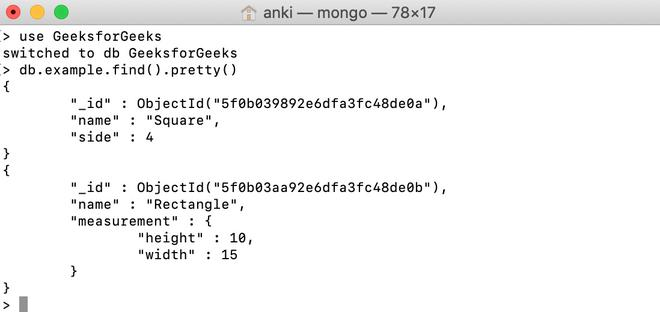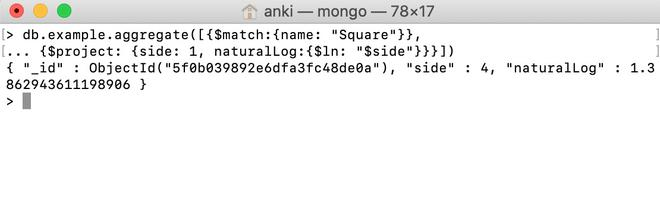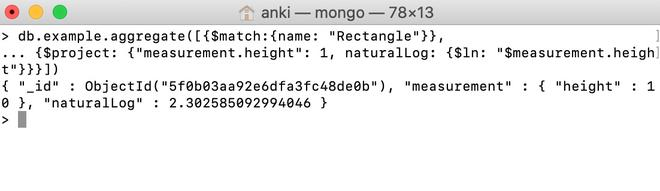GFG App
Open AppBrowser
Continue

MongoDB provides different types of arithmetic expression operators that are used in the aggregation pipeline stages and \$ln operator is one of them. This operator is used to find the natural logarithm of a number and returns the result as a double.

Syntax:

```{ \$ln: <number> }
```

Here, the number is a valid expression until it resolves to a non- negative number.

• If the entered value is null, then this operator will return null.
• If the entered value is NaN, then this operator will return NaN.
• If the entered value is a missing field, then this operator will return null.

Examples:

In the following examples, we are working with:

Database: GeeksforGeeks

Collection: example

Document: two documents that contain the details of the shapes in the form of field-value pairs.Using \$ln operator:

In this example, we are going to find the natural logarithm of the value of the side field in the square document.

```db.example.aggregate([{\$match:{name: "Square"}},
... {\$project: {side: 1, naturalLog:{\$ln: "\$side"}}}])

```Using \$ln operator in the embedded document:

In this example, we are going to find the natural logarithm of the value of the measurement.height field in the rectangle document.

```db.example.aggregate([{\$match:{name: "Rectangle"}},
... {\$project: {"measurement.height": 1,
naturalLog: {\$ln: "\$measurement.height"}}}])

```My Personal Notes arrow_drop_up